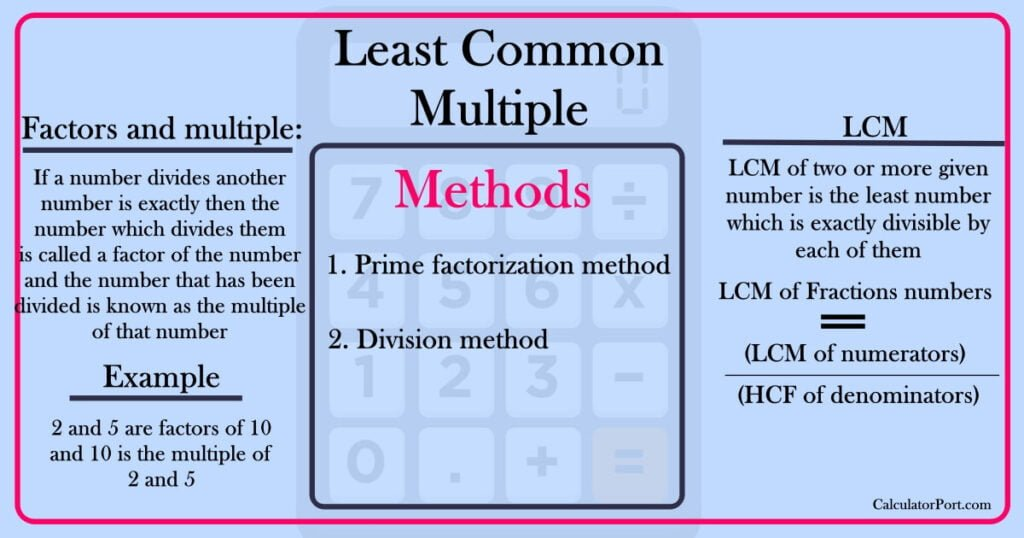# LCM## LCM Calculator of 3 Numbers | Definition | Methods

LCM Calculator of 3 numbers Enter X Enter Y Enter Z Answer: Factors and multiple: If a number divides another number is exactly then the number which divides them is called a factor of the number and the number that has been divided is known as the multiple of that number  Example 2 and 5 …## Least Common Multiple calculator | Definition | Methods

LCM Calculator X Y Answer: Factors and multiple: If a number divides another number is exactly then the number which divides them is called a factor of the number and the number that has been divided is known as the multiple of that number  Example 2 and 5 are factors of 10 and 10 is …

Scroll to Top